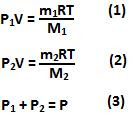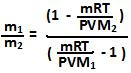## Saturday, January 16, 2010

### Irodov Problem 2.3

The total pressure P in the container is the sum of pressures exerted by gas 1 (P1 ) and gas 2 (P2 ). Let the number of mass of gas 1 be m1 and that of gas 2 be m2. Let the molar mass of gas 1 be M1 and that of gas 2 be M2. So we have,From (1),(2) and (3) we have,The total mass of the gas is the sum of the masses of the individual gases. So we have,From (4) and (5) we can determine the ratios of the masses of the two gases as,1.2.